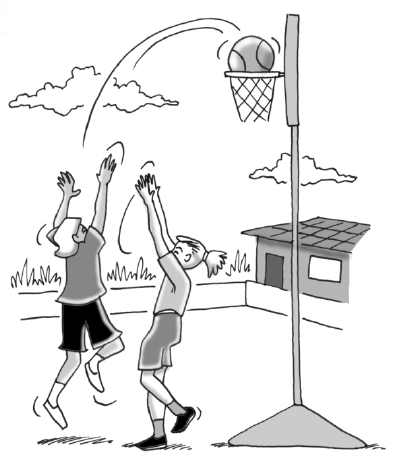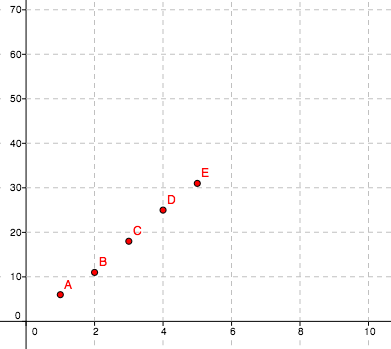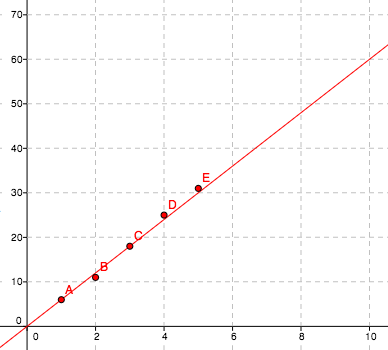### Home > AC > Chapter 9 > Lesson 9.2.3 > Problem9-56

9-56.Stacey is the star of the basketball team. She makes many baskets during each game and could break the record for the most baskets made in one season at her high school. The data for the first five games of this season is below.

Game Number

$1$

$6$

$2$

$11$

$3$

$18$

$4$

$25$

$5$

$31$

1. Plot a graph with these data points.2. Draw a trend line for this data using two carefully selected points that best represent the data.3. Use the equation of your line to predict how many baskets Stacey will make by the end of the season if the season has $15$ games.

$90$ baskets